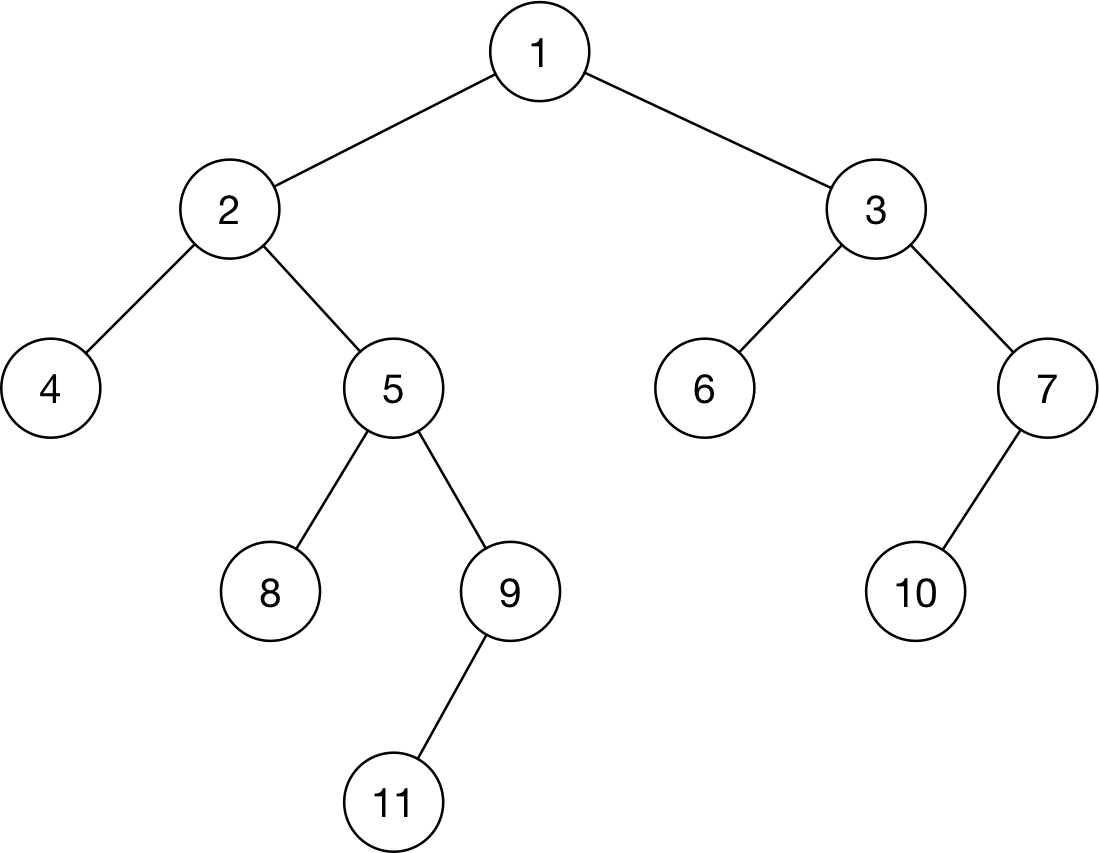# Python 实现二叉树的各种遍历

flyingsmiling

0收藏假设有这么一个二叉树如下：• 前序遍历结果：1, 2, 4, 5, 8, 9, 11, 3, 6, 7, 10
• 中序遍历结果：4, 2, 8, 5, 11, 9, 1, 6, 3, 10, 7
• 后序遍历结果：4, 8, 11, 9, 5, 2, 6, 10, 7, 3, 1

``````class Node(object):
def init(self, value=None, left=None, right=None):
self.value = value
self.left = left
self.right = right

if name == "main":
tree = Node(1, Node(2, Node(4),
Node(5, Node(8),
Node(9, left=Node(11)))),
Node(3, Node(6),
Node(7, left=Node(10))))
``````

``````# 前序遍历（递归）
def pre_deep_func(root):
if root is None:
return
print(root.value, end = ' ')  # print 放到下一行 就是中序遍历，放到最后 就是后序遍历
pre_deep_func(root.left)
pre_deep_func(root.right)

# 前序遍历 生成器
def pre_deep_func(treeNode):
if treeNode:
yield treeNode.value # 同上，调整该行的位置，就实现各种遍历
yield from mid_level(treeNode.left)
yield from mid_level(treeNode.right)``````

1. 由于采取先序遍历，遇到结点就应该访问，下一步就应该沿着树的坐分支下行
2. 但结点的右分支（右子树）还没有访问，因此需要记录，将右子结点入栈。
3. 遇到空树时回溯，取出栈中保存的一个右分支，像一颗二叉树一样遍历它。

``````## 方法一 常规打印
def preorder_nonrec(root):
s = []
while s or root:
while root:  # 沿左分支下行
print(root.value, end = ' ')  # 先处理根数据
s.append(root.right)          # 右分支入栈
root = root.left
root = s.pop()                    #  遇到空树，回溯

## 方法二 通过生成器函数遍历
def preorder_elements(root):
s = []
while s or root:
while root:
s.append(root.right)
yield root.value
root = root.left
root = s.pop()

## 方法三
# 前序遍历（根左右）:模拟压栈过程
# 入栈之前读（根、左），这样出栈时再读右（也是右结点子节点们的根）
def pre_deep_func2(root):
a = []
while a or root:
while root:
print(root.value, end = ' ')
a.append(root)  ## 根入栈
root = root.left
h = a.pop()
root = h.right
``````

``````# 中序遍历（左根右）:模拟压栈过程
# 出栈之后读（左、根），这样出栈后指针变更再读右
def mid_deep_func2(root):
a = []
while a or root:
while root:
a.append(root)
root = root.left
h = a.pop()
print h.value
root = h.right``````

``````# 后序遍历（左右根）:模拟逆序(根右左)存入数组b，然后再数组b逆序输出
# (根右左)与(根左右)类似，入栈a前读（根、右），出栈后指针变更再读左
## 方法 1
def after_deep_func2(root):
a = []
b = []
while a or root:
while root:
b.append(root.value)
a.append(root.left)
root = root.right
root = a.pop()
print(b[::-1])

## 方法2
def after_deep_func2(root):
a = []
b = []
while a or root:
while root:
b.append(root.value)
a.append(root)
root = root.right
h = a.pop()
root = h.left
print b[::-1]
``````

``````## 方法1
ans = [tree.value]
queue = [tree]
while queue:
next_layer = []

for node in queue:
if node.left: next_layer.append(node.left)
if node.right: next_layer.append(node.right)

queue = next_layer

for node in next_layer:
ans.append(node.value)

print(ans)

## 方法2
def level_func(root):
a = []
a.append(root)
while a:帖子
视频
声望
粉丝
最近发布
社区精华内容